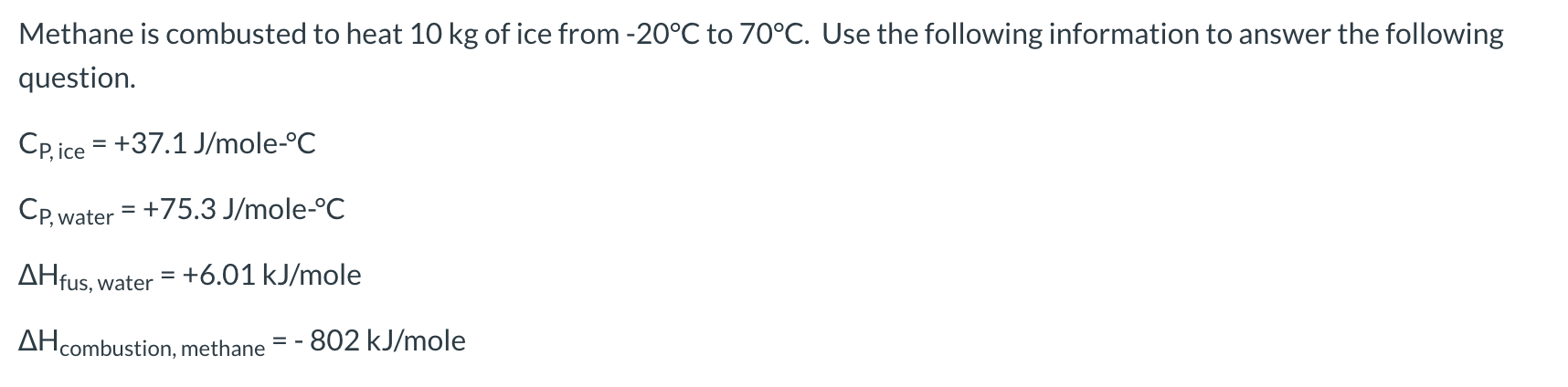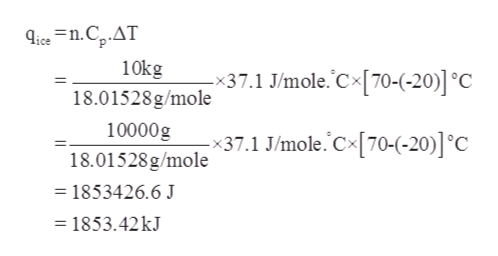# Methane is combusted to heat 10 kg of ice from -20°C to 70°C. Use the following information to answer the followingquestion.CP,ice +37.1 J/mole-°CCp,water 75.3 J/mole-°CAHfus, water= +6.01 kJ/moleAHcombustion, methane802 kJ/mole

Question

Calculate the number of moles of methane needed to complete the heating process.help_outlineImage TranscriptioncloseMethane is combusted to heat 10 kg of ice from -20°C to 70°C. Use the following information to answer the following question. CP,ice +37.1 J/mole-°C Cp,water 75.3 J/mole-°C AHfus, water = +6.01 kJ/mole AHcombustion, methane 802 kJ/mole fullscreen
check_circleExpert Solution
Step 1

Given information:

Mass of ice = 10 kg

Initial temperature = -20 °C

Final temperature = 70 °C

Cp,ice = + 37.1 J/mole.°C

Cp,water = + 75.3 J/mole.°C

ΔHfus, water = + 6.01 kJ/mole

ΔHcombustion, methane = -802 kJ/mole

Step 2

The amount of heat required to raise the temperature by 1°C is known as heat capacity. The heat capacity at constant pressure is represented by Cp.

Step 3

The amount of heat released or absorbed can be expressed in terms of heat capacity and temperature chan...help_outlineImage Transcriptioncloseice n.Cp.AT 10kg 18.01528g/mole -x37.1 J/mole. Cx[70-(-20)] °C 10000g x37.1 J/mole. C70-(-20)] °C 18.01528g/mole = 1853426.6 J = 1853.42 kJ fullscreen

### Want to see the full answer?

See Solution

#### Want to see this answer and more?

Solutions are written by subject experts who are available 24/7. Questions are typically answered within 1 hour*

See Solution
*Response times may vary by subject and question
Tagged in

### Chemistry